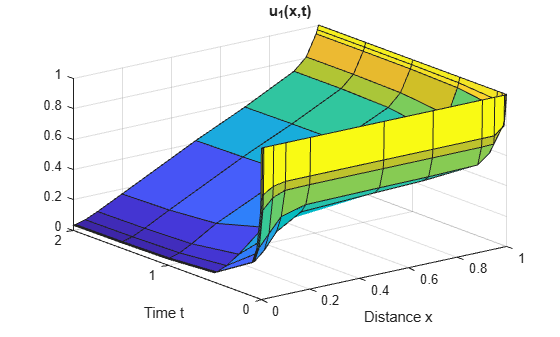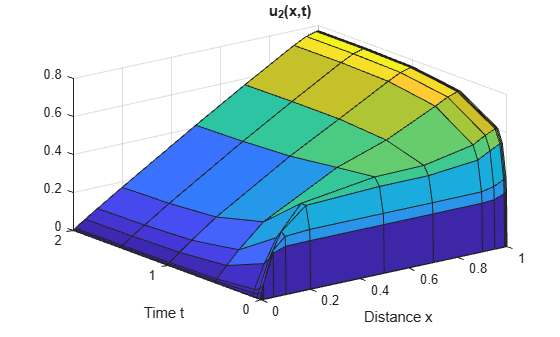# Solve System of PDEs

This example shows how to formulate, compute, and plot the solution to a system of two partial differential equations.

Consider the system of PDEs

`$\frac{\partial {\mathit{u}}_{1}}{\partial \mathit{t}}=0.024\frac{{\partial }^{2}{\mathit{u}}_{1}}{\partial {\mathit{x}}^{2}}-\mathit{F}\left({\mathit{u}}_{1}-{\mathit{u}}_{2}\right),$`

`$\frac{\partial {\mathit{u}}_{2}}{\partial \mathit{t}}=0.170\frac{{\partial }^{2}{\mathit{u}}_{2}}{\partial {\mathit{x}}^{2}}+\mathit{F}\left({\mathit{u}}_{1}-{\mathit{u}}_{2}\right).$`

(The function $\mathit{F}\left(\mathit{y}\right)={\mathit{e}}^{5.73\mathit{y}}-{\mathit{e}}^{-11.46\mathit{y}}$ is used as a shorthand.)

The equation holds on the interval $0\le \mathit{x}\le 1$ for times $\mathit{t}\ge 0$. The initial conditions are

`${\mathit{u}}_{1}\left(\mathit{x},0\right)=1,$`

`${\mathit{u}}_{2}\left(\mathit{x},0\right)=0.$`

The boundary conditions are

`$\begin{array}{l}\frac{\partial }{\partial \mathit{x}}{\mathit{u}}_{1}\left(0,\mathit{t}\right)=0,\\ {\text{\hspace{0.17em}}\text{\hspace{0.17em}}\text{\hspace{0.17em}}\text{\hspace{0.17em}}\text{\hspace{0.17em}}\text{\hspace{0.17em}}\mathit{u}}_{2}\left(0,\mathit{t}\right)=0,\\ \frac{\partial }{\partial \mathit{x}}{\mathit{u}}_{2}\left(1,\mathit{t}\right)=0,\\ {\text{\hspace{0.17em}}\text{\hspace{0.17em}}\text{\hspace{0.17em}}\text{\hspace{0.17em}}\text{\hspace{0.17em}}\text{\hspace{0.17em}}\mathit{u}}_{1}\left(1,\mathit{t}\right)=1.\end{array}$`

To solve this equation in MATLAB®, you need to code the equation, the initial conditions, and the boundary conditions, then select a suitable solution mesh before calling the solver `pdepe`. You either can include the required functions as local functions at the end of a file (as done here), or save them as separate, named files in a directory on the MATLAB path.

### Code Equation

Before you can code the equation, you need to make sure that it is in the form that the `pdepe` solver expects:

`$\mathit{c}\left(\mathit{x},\mathit{t},\mathit{u},\frac{\partial \mathit{u}}{\partial \mathit{x}}\right)\frac{\partial \mathit{u}}{\partial \mathit{t}}={\mathit{x}}^{-\mathit{m}}\frac{\partial }{\partial \mathit{x}}\left({\mathit{x}}^{\mathit{m}}\mathit{f}\left(\mathit{x},\mathit{t},\mathit{u},\frac{\partial \mathit{u}}{\partial \mathit{x}}\right)\right)+\mathit{s}\left(\mathit{x},\mathit{t},\mathit{u},\frac{\partial \mathit{u}}{\partial \mathit{x}}\right).$`

In this form, the PDE coefficients are matrix-valued and the equation becomes

`$\left[\begin{array}{cc}1& 0\\ 0& 1\end{array}\right]\frac{\partial }{\partial \mathit{t}}\left[\begin{array}{c}{\mathit{u}}_{1}\\ {\mathit{u}}_{2}\end{array}\right]=\frac{\partial }{\partial \mathit{x}}\left[\begin{array}{c}0.024\frac{\partial {\mathit{u}}_{1}}{\partial \mathit{x}}\\ 0.170\frac{\partial {\mathit{u}}_{2}}{\partial \mathit{x}}\end{array}\right]+\left[\begin{array}{c}-\mathit{F}\left({\mathit{u}}_{1}-{\mathit{u}}_{2}\right)\\ \mathit{F}\left({\mathit{u}}_{1}-{\mathit{u}}_{2}\right)\end{array}\right].$`

So the values of the coefficients in the equation are

`$\mathit{m}=0$`

$\mathit{c}\left(\mathit{x},\mathit{t},\mathit{u},\frac{\partial \mathit{u}}{\partial \mathit{x}}\right)=\left[\begin{array}{c}1\\ 1\end{array}\right]$ (diagonal values only)

`$\mathit{f}\left(\mathit{x},\mathit{t},\mathit{u},\frac{\partial \mathit{u}}{\partial \mathit{x}}\right)=\left[\begin{array}{c}0.024\frac{\partial {\mathit{u}}_{1}}{\partial \mathit{x}}\\ 0.170\frac{\partial {\mathit{u}}_{2}}{\partial \mathit{x}}\end{array}\right]$`

`$\mathit{s}\left(\mathit{x},\mathit{t},\mathit{u},\frac{\partial \mathit{u}}{\partial \mathit{x}}\right)=\left[\begin{array}{c}-\mathit{F}\left({\mathit{u}}_{1}-{\mathit{u}}_{2}\right)\\ \mathit{F}\left({\mathit{u}}_{1}-{\mathit{u}}_{2}\right)\end{array}\right]$`

Now you can create a function to code the equation. The function should have the signature `[c,f,s] = pdefun(x,t,u,dudx)`:

• `x` is the independent spatial variable.

• `t` is the independent time variable.

• `u` is the dependent variable being differentiated with respect to `x` and `t`. It is a two-element vector where `u(1)` is ${\mathit{u}}_{1}\left(\mathit{x},\mathit{t}\right)$ and `u(2)` is ${\mathit{u}}_{2}\left(\mathit{x},\mathit{t}\right)$.

• `dudx` is the partial spatial derivative $\partial \mathit{u}/\partial \mathit{x}$. It is a two-element vector where `dudx(1)` is $\partial {\mathit{u}}_{1}/\partial \mathit{x}$ and `dudx(2)` is $\partial {\mathit{u}}_{2}/\partial \mathit{x}$.

• The outputs `c`, `f`, and `s` correspond to coefficients in the standard PDE equation form expected by `pdepe`.

As a result, the equations in this example can be represented by the function:

```function [c,f,s] = pdefun(x,t,u,dudx) c = [1; 1]; f = [0.024; 0.17] .* dudx; y = u(1) - u(2); F = exp(5.73*y)-exp(-11.47*y); s = [-F; F]; end ```

(Note: All functions are included as local functions at the end of the example.)

### Code Initial Conditions

Next, write a function that returns the initial condition. The initial condition is applied at the first time value and provides the value of $\mathit{u}\left(\mathit{x},{\mathit{t}}_{0}\right)$ for any value of x. The number of initial conditions must equal the number of equations, so for this problem there are two initial conditions. Use the function signature `u0 = pdeic(x)` to write the function.

The initial conditions are

`${\mathit{u}}_{1}\left(\mathit{x},0\right)=1,$`

`${\mathit{u}}_{2}\left(\mathit{x},0\right)=0.$`

The corresponding function is

```function u0 = pdeic(x) u0 = [1; 0]; end ```

### Code Boundary Conditions

Now, write a function that evaluates the boundary conditions

`$\begin{array}{l}\frac{\partial }{\partial \mathit{x}}{\mathit{u}}_{1}\left(0,\mathit{t}\right)=0,\\ {\text{\hspace{0.17em}}\text{\hspace{0.17em}}\text{\hspace{0.17em}}\text{\hspace{0.17em}}\text{\hspace{0.17em}}\text{\hspace{0.17em}}\mathit{u}}_{2}\left(0,\mathit{t}\right)=0,\\ \frac{\partial }{\partial \mathit{x}}{\mathit{u}}_{2}\left(1,\mathit{t}\right)=0,\\ {\text{\hspace{0.17em}}\text{\hspace{0.17em}}\text{\hspace{0.17em}}\text{\hspace{0.17em}}\text{\hspace{0.17em}}\text{\hspace{0.17em}}\mathit{u}}_{1}\left(1,\mathit{t}\right)=1.\end{array}$`

For problems posed on the interval $\mathit{a}\le \mathit{x}\le \mathit{b}$, the boundary conditions apply for all $\mathit{t}$ and either $\mathit{x}=\mathit{a}$ or $\mathit{x}=\mathit{b}$. The standard form for the boundary conditions expected by the solver is

`$\mathit{p}\left(\mathit{x},\mathit{t},\mathit{u}\right)+\mathit{q}\left(\mathit{x},\mathit{t}\right)\mathit{f}\left(\mathit{x},\mathit{t},\mathit{u},\frac{\partial \mathit{u}}{\partial \mathit{x}}\right)=0.$`

Written in this form, the boundary conditions for the partial derivatives of $\mathit{u}$ need to be expressed in terms of the flux $\mathit{f}\left(\mathit{x},\mathit{t},\mathit{u},\frac{\partial \mathit{u}}{\partial \mathit{x}}\right)$. So the boundary conditions for this problem are

For $\mathit{x}=0$, the equation is

`$\left[\begin{array}{c}0\\ {\mathit{u}}_{2}\end{array}\right]+\left[\begin{array}{c}1\\ 0\end{array}\right]\cdot \left[\begin{array}{c}0.024\frac{\partial {\mathit{u}}_{1}}{\partial \mathit{x}}\\ 0.170\frac{\partial {\mathit{u}}_{2}}{\partial \mathit{x}}\end{array}\right]=0.$`

The coefficients are:

`${\mathit{p}}_{\mathit{L}}\left(\mathit{x},\mathit{t},\mathit{u}\right)=\left[\begin{array}{c}0\\ {\mathit{u}}_{2}\end{array}\right],$`

`${\mathit{q}}_{\mathit{L}}\left(\mathit{x},\mathit{t}\right)=\left[\begin{array}{c}1\\ 0\end{array}\right].$`

Likewise, for $\mathit{x}=1$ the equation is

`$\left[\begin{array}{c}{\mathit{u}}_{1}-1\\ 0\end{array}\right]+\left[\begin{array}{c}0\\ 1\end{array}\right]\cdot \left[\begin{array}{c}0.024\frac{\partial {\mathit{u}}_{1}}{\partial \mathit{x}}\\ 0.170\frac{\partial {\mathit{u}}_{2}}{\partial \mathit{x}}\end{array}\right]=0.$`

The coefficients are:

`${\mathit{p}}_{\mathit{R}}\left(\mathit{x},\mathit{t},\mathit{u}\right)=\left[\begin{array}{c}{\mathit{u}}_{1}-1\\ 0\end{array}\right],$`

`${\mathit{q}}_{\mathit{R}}\left(\mathit{x},\mathit{t}\right)=\left[\begin{array}{c}0\\ 1\end{array}\right].$`

The boundary function should use the function signature `[pl,ql,pr,qr] = pdebc(xl,ul,xr,ur,t)`:

• The inputs `xl` and `ul` correspond to $\mathit{u}$ and $\mathit{x}$ for the left boundary.

• The inputs `xr` and `ur` correspond to $\mathit{u}$ and $\mathit{x}$ for the right boundary.

• `t` is the independent time variable.

• The outputs `pl` and `ql` correspond to ${\mathit{p}}_{\mathit{L}}\left(\mathit{x},\mathit{t},\mathit{u}\right)$ and ${\mathit{q}}_{\mathit{L}}\left(\mathit{x},\mathit{t}\right)$ for the left boundary ($\mathit{x}=0$ for this problem).

• The outputs `pr` and `qr` correspond to ${\mathit{p}}_{\mathit{R}}\left(\mathit{x},\mathit{t},\mathit{u}\right)$ and ${\mathit{q}}_{\mathit{R}}\left(\mathit{x},\mathit{t}\right)$ for the right boundary ($\mathit{x}=1$ for this problem).

The boundary conditions in this example are represented by the function:

```function [pl,ql,pr,qr] = pdebc(xl,ul,xr,ur,t) pl = [0; ul(2)]; ql = [1; 0]; pr = [ur(1)-1; 0]; qr = [0; 1]; end ```

### Select Solution Mesh

The solution to this problem changes rapidly when $\mathit{t}$ is small. Although `pdepe` selects a time step that is appropriate to resolve the sharp changes, to see the behavior in the output plots you need to select appropriate output times. For the spatial mesh, there are boundary layers in the solution at both ends of $0\le \mathit{x}\le 1$, so you need to specify mesh points there to resolve the sharp changes.

```x = [0 0.005 0.01 0.05 0.1 0.2 0.5 0.7 0.9 0.95 0.99 0.995 1]; t = [0 0.005 0.01 0.05 0.1 0.5 1 1.5 2];```

### Solve Equation

Finally, solve the equation using the symmetry $\mathit{m}$, the PDE equation, the initial conditions, the boundary conditions, and the meshes for $\mathit{x}$ and $\mathit{t}$.

```m = 0; sol = pdepe(m,@pdefun,@pdeic,@pdebc,x,t);```

`pdepe` returns the solution in a 3-D array `sol`, where `sol(i,j,k)` approximates the `k`th component of the solution ${\mathit{u}}_{\mathit{k}}$ evaluated at `t(i)` and `x(j)`. Extract each solution component into a separate variable.

```u1 = sol(:,:,1); u2 = sol(:,:,2);```

### Plot Solution

Create surface plots of the solutions for ${\mathit{u}}_{1}$ and ${\mathit{u}}_{2}$ plotted at the selected mesh points for $\mathit{x}$ and $\mathit{t}$.

```surf(x,t,u1) title('u_1(x,t)') xlabel('Distance x') ylabel('Time t')``````surf(x,t,u2) title('u_2(x,t)') xlabel('Distance x') ylabel('Time t')```### Local Functions

Listed here are the local helper functions that the PDE solver `pdepe` calls to calculate the solution. Alternatively, you can save these functions as their own files in a directory on the MATLAB path.

```function [c,f,s] = pdefun(x,t,u,dudx) % Equation to solve c = [1; 1]; f = [0.024; 0.17] .* dudx; y = u(1) - u(2); F = exp(5.73*y)-exp(-11.47*y); s = [-F; F]; end % --------------------------------------------- function u0 = pdeic(x) % Initial Conditions u0 = [1; 0]; end % --------------------------------------------- function [pl,ql,pr,qr] = pdebc(xl,ul,xr,ur,t) % Boundary Conditions pl = [0; ul(2)]; ql = [1; 0]; pr = [ur(1)-1; 0]; qr = [0; 1]; end % ---------------------------------------------```